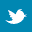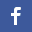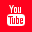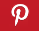Completely Solved C, C++ Programs Assignment.Quick Search Database Videos Tutorials Ebooks Forums FAQ Aboutus Household Industrial Manufacturing Service Shopping Transportation### Dynamic Memory Allocation

 Filed Under:

 Dynamic Memory Allocation:
Write a program in C to read and display integer, float, char, double number

# Write a program in C to sum of two integer numbers

Write a program in C to larger of two integer numbers

# Write a program in C to read and display text using dynamic allocation

Write a program in C to read N characters

# Write a program in C to merge two arrays

Write a program in C to read and display biodata using structures

# Write a program in C to read and display integer array using functions

Write a program in C to read and display biodata using functions

# Write a program in C to print the sum of two 3 x 3 Matrix Write a program in C to print the product of two 3 x 3 Matrix

Write a program in C to read and display a N x N Matrix
Write a program in C to transpose the N x N Matrix

# Write a program in C to print the sum of two N x N Matrix

Write a program in C to print the product of two N x N Matrix
Write a program in C to read and display a M x N Matrix
Write a program in C to transpose of M x N Matrix
Write a program in C to print the sum of two M x N Matrix
Write a program in C to print the product of two M x N Matrix